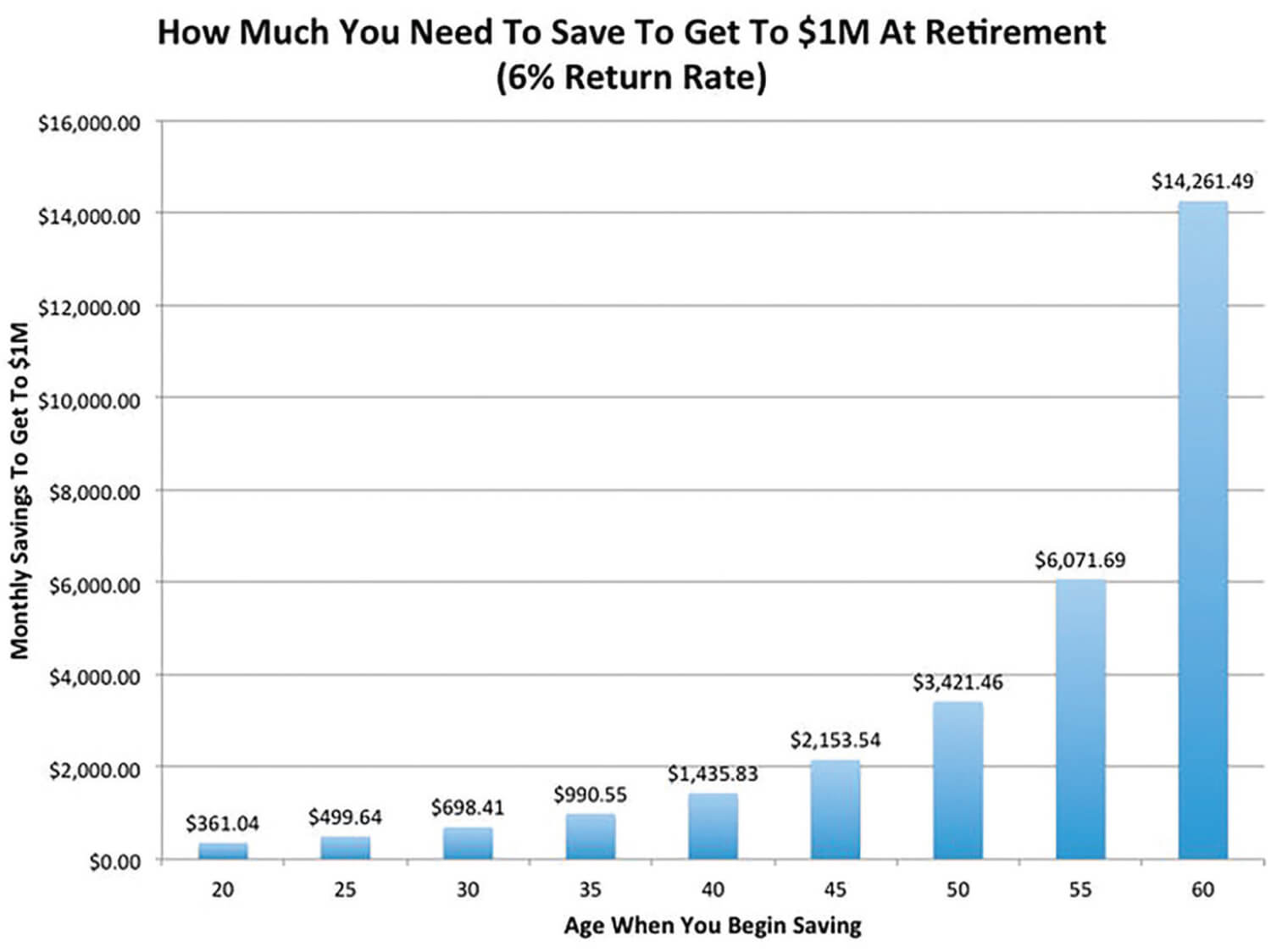Compound Interest is the 8th Wonder of the World

According to Einstein, compound interest is the 8th wonder of the world.

WHAT IS COMPOUND INTEREST?

Compound interest is the addition of interest to the principal sum of a loan or deposit, or in other words, interest on interest. It is the result of reinvesting interest, rather than paying it out, so that interest in the next period is then earned on the principal sum plus previously accumulated interest.The formula for compound interest, including principal sum, is: A = P (1 + r/n) (nt)

EXAMPLE:
If an amount of \$5,000 is deposited into a savings account at an annual interest rate of 5%, compounded monthly, the value of the investment after 10 years can be calculated as follows…

P (Principal or invested amount) = 5000
r (annual rate) = 5/100 = 0.05 (decimal)
n (number of times compounded) = 12
t (time the money is invested) = 10

A = 5000 (1 + 0.05 / 12)
(12 * 10) = 8235.05.

So, the investment balance after 10 years is \$8,235.05.

THE RULE OF 72

To find the number of years required to double your money at a given interest rate, divide the interest rate into 72. Example: How long it will take to double your money at 8% interest? 9 years (divide 8 into 72).